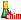﻿ Combustion reactions of alkanesSearch:

# Combustion reactions of alkanes

## Complete combustion

Complete combustion is combustion in the presence of excess air

### Example: methane1. Methane 2. Air 3. Production of carbon dioxide and water vapor $CH_4 + 2O_2 \; \rightarrow \; CO_2 + 2H_2O$

### Linear or branched alkanes

$2 \; C_nH_{2n + 2} + (3n + 1) \; O_2 \; \rightarrow \; 2n \; CO_2 + (2n + 2) \; H_2O$

Look at →       a video that shows the complete combustion of butane and tests the products of this reaction.

## Incomplete combustion

Incomplete combustion is combustion in air in default.

### Example: methane1. Methane 2. No air inlet 3. Carbon production (which colors the yellow flame) and water vapor $CH_4 + O_2 \; \rightarrow \; C + 2H_2O$

### Linear or branched alkanes

$2 \; C_nH_{2n + 2} + (n + 1) \; O_2 \; \rightarrow \; 2n \; C + (2n + 2) \; H_2O$

Look at →       a video that shows the incomplete combustion of butane and the products of this reaction.